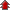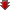Home Board Paper Solutions Text Book Solutions Articles NCERT Exemplar Solutions

# Coordination Compounds### Exercise 1

•  Q1 Write the formulas for the following coordination compounds: (i) Tetraamminediaquacobalt (III) chloride (ii) Potassium tetracyanonickelate(II) (iii) Tris(ethane-1,2-diamine) chromium(III) chloride (iv) Amminebromidochloridonitrito-N-platinate(II) (v) Dichloridobis(ethane-1,2-diamine)platinum(IV) nitrate (vi) Iron(III) hexacyanoferrate(II)">Write the formulas for the following coordination compounds: (i) Tetraamminediaquacobalt (III) chloride (ii) Potassium tetracyanonickelate(II) (iii) Tris(ethane-1,2-diamine) chromium(III) chloride (iv) Amminebromidochloridonitrito-N-platinate(II) (v) Dichloridobis(ethane-1,2-diamine)platinum(IV) nitrate (vi) Iron(III) hexacyanoferrate(II) Q2 Write the IUPAC names of the following coordination compounds:   (i) [Co(NH3)6]Cl3 (ii) [Co(NH3)5Cl]Cl2 (iii) K3[Fe(CN)6] (iv) K3[Fe(C2O4)3] (v) K2[PdCl4] (vi) [Pt(NH3)2Cl(NH2CH3)]Cl Q3 Indicate the types of isomerism exhibited by the following complexes and draw the structures for these isomers: (i) K[Cr(H2O)2(C2O4)2 (ii) [Co(en)3]Cl3 (iii) [Co(NH3)5(NO2)](NO3)2 (iv) [Pt(NH3)(H2O)Cl2] Q4 Give evidence that [Co(NH3)5Cl]SO4 and [Co(NH3)5SO4]Cl are ionization isomers. Q5 Explain on the basis of valence bond theory that [Ni(CN)4]2- ion with square planar structure is diamagnetic and the [Ni(CN)4]2- ion with tetrahedral geometry is paramagnetic. Q6 [NiCl4]2- is paramagnetic while [Ni(CO)4] is diamagnetic though both are tetrahedral. Why? Q7 [Fe(H2O)6]3+ is strongly paramagnetic whereas [Fe(CN)6]3- is weakly paramagnetic. Explain. Q8 Explain [Co(NH3)6]3+ is an inner orbital complex whereas [Ni(NH3)6]2+ is an outer orbital complex. Q9 Predict the number of unpaired electrons in the square planar [Pt(CN)4]2- ion. Q10 The hexaquo manganese(II) ion contains five unpaired electrons, while the hexacyanoion contains only one unpaired electron. Explain using Crystal Field Theory.">The hexaquo manganese(II) ion contains five unpaired electrons, while the hexacyanoion contains only one unpaired electron. Explain using Crystal Field Theory. Q11 Calculate the overall complex dissociation equilibrium constant for the Cu(NH3)42+ ion, given that  β4 for this complex is 2.1 x 1013.">Calculate the overall complex dissociation equilibrium constant for the Cu(NH3)42+ ion, given that  β4 for this complex is 2.1 x 1013.### Exercise 2

•  Q1 Explain the bonding in coordination compounds in terms of Werner’s postulates. Q2 FeSO4 solution mixed with (NH4)2SO4 solution in 1:1 molar ratio gives the test of Fe2+ ion but CuSO4 solution mixed with aqueous ammonia in 1:4 molar ratio does not give the test of Cu2+ ion. Explain why?">FeSO4 solution mixed with (NH4)2SO4 solution in 1:1 molar ratio gives the test of Fe2+ ion but CuSO4 solution mixed with aqueous ammonia in 1:4 molar ratio does not give the test of Cu2+ ion. Explain why? Q3 Explain with two examples each of the following: coordination entity, ligand, coordination number, coordination polyhedron, homoleptic and heteroleptic.">Explain with two examples each of the following: coordination entity, ligand, coordination number, coordination polyhedron, homoleptic and heteroleptic. Q4 What is meant by unidentate, didentate and ambidentate ligands? Give two examples for each. Q5 Specify the oxidation numbers of the metals in the following coordination entities: (i) [Co(H2O)(CN)(en)2]2+ (ii) [CoBr2(en)2]+ (iii) [PtCl4]2- (iv) K3[Fe(CN)6] (v) [Cr(NH3)3Cl3] Q6 Using IUPAC norms write the formulas for the following: (i) Tetrahydroxozincate(II) (ii) Potassium tetrachloridopalladate(II) (iii) Diamminedichloridoplatinum(II) (iv) Potassium tetracyanonickelate(II) (v) Pentaamminenitrito-O-cobalt(III) (vi) Hexaamminecobalt(III) sulphate (vii) Potassium tri(oxalato)chromate(III) (viii) Hexaammineplatinum(IV) (ix) Tetrabromidocuprate(II) (x) Pentaamminenitrito-N-cobalt(III) Q7 Using IUPAC norms write the systematic names of the following: (i) [Co(NH3)6]Cl3 (ii) [Pt(NH3)2Cl(NH2CH3)]Cl (iii) [Ti(H2O)6]3+ (iv) [Co(NH3)4Cl(NO2)]Cl (v) [Mn(H2O)6]2+ (vi) [NiCl4]2- (vii) [Ni(NH3)6]Cl2 (viii) [Co(en)3]3+ (ix) [Ni(CO)4] Q8 List various types of isomerism possible for coordination compounds, giving an example of each.">List various types of isomerism possible for coordination compounds, giving an example of each. Q9 How many geometrical isomers are possible in the following coordination entities? (i) [Cr(C2O4)3]3- (ii) [Co(NH3)3Cl3] Q10 Draw the structures of optical isomers of: (i) [Cr(C2O4)3]3- (ii) [PtCl2(en)2]2+ (iii) [Cr(NH3)2Cl2(en)]+ Q11 Draw all the isomers (geometrical and optical) of: (i) [CoCl2(en)2]+ (ii) [Co(NH3)Cl(en)2]2+ (iii) [Co(NH3)2Cl2(en)]+ Q12 Write all the geometrical isomers of [Pt(NH3)(Br)(Cl)(py)] and how many of these will exhibit optical isomers?">Write all the geometrical isomers of [Pt(NH3)(Br)(Cl)(py)] and how many of these will exhibit optical isomers? Q13 Aqueous copper sulphate solution (blue in colour) gives: (i) a green precipitate with aqueous potassium fluoride, and (ii) a bright green solution with aqueous potassium chloride Explain these experimental results.">Aqueous copper sulphate solution (blue in colour) gives: (i) a green precipitate with aqueous potassium fluoride, and (ii) a bright green solution with aqueous potassium chloride Explain these experimental results. Q14 What is the coordination entity formed when excess of aqueous KCN is added to an aqueous solution of copper sulphate? Why is it that no precipitate of copper sulphide is obtained when H2S(g) is passed through this solution?">What is the coordination entity formed when excess of aqueous KCN is added to an aqueous solution of copper sulphate? Why is it that no precipitate of copper sulphide is obtained when H2S(g) is passed through this solution? Q15 Discuss the nature of bonding in the following coordination entities on the basis of valence bond theory: (i) [Fe(CN)6]4- (ii) [FeF6]3- (iii) [Co(C2O4)3]3- (iv) [CoF6]3- Q16 Draw figure to show the splitting of d orbitals in an octahedral crystal field.">Draw figure to show the splitting of d orbitals in an octahedral crystal field. Q17 What is spectrochemical series? Explain the difference between a weak field ligand and a strong field ligand.">What is spectrochemical series? Explain the difference between a weak field ligand and a strong field ligand. Q18 What is crystal field splitting energy? How does the magnitude of Δo decide the actual configuration of d-orbitals in a coordination entity?">What is crystal field splitting energy? How does the magnitude of Δo decide the actual configuration of d-orbitals in a coordination entity? Q19 [Cr(NH3)6]3+ is paramagnetic while [Ni(CN)4]2- is diamagnetic.  Explain why? Q20 A solution of [Ni(H2O)6]2+ is green but a solution of [Ni(CN)4]2- is colourless. Explain. Q21 [Fe(CN)6]4- and [Fe(H2O)6]2+ are of different colours in dilute solutions. Why? Q22 Discuss the nature of bonding in metal carbonyls. Q23 Give the oxidation state, d-orbital occupation and coordination number of the central metal ion in the following complexes: (i) K3[Co(C2O4)3] (ii) cis-[Cr(en)2Cl2]Cl (iii) (NH4)2[CoF4] (iv) [Mn(H2O)6]SO4 ">Give the oxidation state, d-orbital occupation and coordination number of the central metal ion in the following complexes: (i) K3[Co(C2O4)3] (ii) cis-[Cr(en)2Cl2]Cl (iii) (NH4)2[CoF4] (iv) [Mn(H2O)6]SO4 Q24 Write down the IUPAC name for each of the following complexes and indicate the oxidation state, electronic configuration and coordination number. Also give stereochemistry and magnetic moment of the complex: (i) K[Cr(H2O)2(C2O4)2].3H2O (ii) [Co(NH3)5Cl]Cl2 (iii) CrCl3(py)3 (iv) Cs[FeCl4] (v) K4[Mn(CN)6]">Write down the IUPAC name for each of the following complexes and indicate the oxidation state, electronic configuration and coordination number. Also give stereochemistry and magnetic moment of the complex: (i) K[Cr(H2O)2(C2O4)2].3H2O (ii) [Co(NH3)5Cl]Cl2 (iii) CrCl3(py)3 (iv) Cs[FeCl4] (v) K4[Mn(CN)6] Q25 What is meant by stability of a coordination compound in solution? State the factors which govern stability of complexes.">What is meant by stability of a coordination compound in solution? State the factors which govern stability of complexes. Q26 What is meant by the chelate effect? Give an example. Q27 Discuss briefly giving an example in each case the role of coordination compounds in: (i) biological system (ii) medicinal chemistry (iii) analytical chemistry (iv) extraction/metallurgy of metals">Discuss briefly giving an example in each case the role of coordination compounds in: (i) biological system (ii) medicinal chemistry (iii) analytical chemistry (iv) extraction/metallurgy of metals Q28 How many ions are produced from the complex Co(NH3)6Cl2 in solution? (i) 6 (ii) 4 (iii) 3 (iv) 2 Q29 Amongst the following ions which one has the highest magnetic moment value? (i) [Cr(H2O)6]3+ (ii) [Fe(H2O)6]2+ (iii) [Zn(H2O)6]2+ Q30 The oxidation number of cobalt in K[Co(CO)4] is (i) +1 (ii) +3 (iii) -1 (iv) -3 Q31 Amongst the following, the most stable complex is (i) [Fe(H2O)6]3+ (ii) [Fe(NH3)6]3+ (iii) [Fe(C2O4)3]3- (iv) [FeCl6]3- Q32 What will be the correct order for the wavelengths of absorption in the visible region for the following: [Ni(NO2)6]4-,      [Ni(NH3)6]2+,      [Ni(H2O)6]2+

## Recently Viewed Questions of Class 12th chemistry

• NCERT Chapter